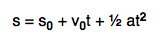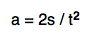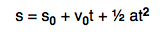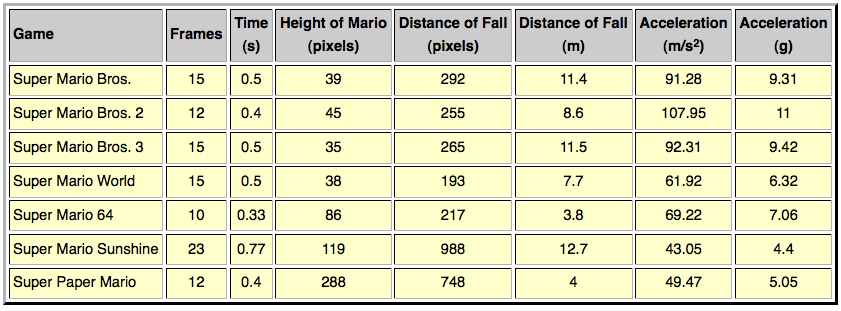# ACCELERATION DUE TO GRAVITY: SUPER MARIO BROTHERSAbstract
The purpose of this analysis is to determine the evolution of gravity in the Mario video game series as video game hardware increases.

Introduction
Gravity is force which is responsible for keeping us on the ground. It is also the force that prohibits us from jumping 50 feet in the air. However, in Mario’s world, gravity does not quite work that way. Mario is able to jump 5 times his height and fall with accelerations that would be deadly to humans.

We will find Mario’s acceleration due to gravity by using the formula:where s is the distance he falls, s0 is his initial distance, which is 0, v0 is his initial vertical velocity, which is also 0, a is his acceleration due to gravity, and t is the time it takes for him to fall. When we solve this formula for a, we get:Procedure
1. Record video clips of Mario falling from a ledge in the following games:

• Super Mario Bros 1, 2 & 3, for NES
• Super Mario World for SNE
• Super Mario 64 for N64
• Super Mario Sunshine GCN
• Super Paper Mario for Wii

2. Watch the clip in Quicktime Video Player and use the frame by frame option to determine the number of frames it took Mario to fall. Also using Quicktime, the FPS, or frames per second, of each video must be found.

3. Take a screen shot of Mario standing next to the ledge. This Screen shot will be used to determine the distance of the fall.

Analysis
First, you must find the time it took Mario to fall from the edge of the ledge to the ground in each game. To do this, we opened each clip in Quicktime movie player, and using the frame by frame option, found the total number of frames it took Mario to fall. We then used the formula:To find the time of each of Mario’s falls. Once we knew the time, we needed to figure out the distance Mario fell in each game. We used a screen shot of Mario next to the ledge he fell from in each game, and found the height of Mario and the ledge in pixels. According to Wikipedia, Mario is “a little over five feet tall.”, so we used 5 feet, or 1.524 meters, as Mario’s height. We used the formula:Once we had the distance Mario fell in each instance, we were able to use the formulato find Mario’s acceleration in each game. Mario was in free fall in each case, so this acceleration was equal to gravity. His initial velocity was 0, as was his initial position. Our results in m/s2 as well as in multiples of g are outlined in the table below.(Click on the table to see full size image)

Finally, we graphed the acceleration due to gravity in each game as the bit rate of the graphics processor increased. Since Super Mario Bros. 1, 2, and 3 were from the same console, we took an average of the three values. Also, the Nintendo Wii never clearly defined its bit rate, but sources say that it is 96 Bits, which is actually less than that of the Nintendo GameCube. As for the other systems, the NES is an 8 Bit system, the SNES is 16 bit, the N64 is 64 Bit, and the GCN is 128 Bit. We set a power fit to this graph, and the result is shown below.Conclusion
We determined that, generally speaking, the gravity in each Mario game, as game hardware has increased, is getting closer to the true value of gravity on earth of 9.8 m/s2. However, gravity, even on the newest consoles, is still extreme. According to Wikipedia, a typical person can withstand 5 g before losing consciousness, and all but the very latest of Mario games have gravity greater than this. Also, with gravity that great, it is a wonder Mario can perform such feats as leaping almost 5 times his own body height!

Sources of Error
The primary source of error in this experiment would be the assumption that Mario is 5 feet tall, and that his height stays constant in each game. In most Mario games, he can become bigger by consuming mushrooms or other powerup objects, and the 5 foot height may be referring to this state. Also, in the 3D Mario games, the camera angle was always angled down, so when measuring the height of Mario and the ledge, this angle caused the measured distance to be different than the actual distance.